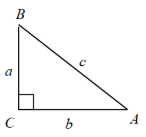### Home > CCA2 > Chapter Ch12 > Lesson 12.1.4 > Problem12-76

12-76.

Using the triangle at right, find:

This problem is similar to problem 12-67. Refer back to that problem if you need some help.1. $\sec B$

What trigonometric ratio is sec$(x)$ a reciprocal of?
What parts of the triangle are used in that ratio?

1. $\tan A$

Remember that tan$(x)$ is equal to opposite over adjacent.

$\frac{a}{b}$

1. $\cot A$

This is related to part (b). How?

1. $\csc A$

What trigonometric ratio is csc$(x)$ a reciprocal of?

$\frac{c}{a}$